# Sequence to sequence model - class review

Last updated on：3 months ago

Sequences are a regular type of dataset for deep learning. Let’s see how to feed them into our RNN models.

# Sequence to sequence model

## Basic model

Encoder network, decoder network.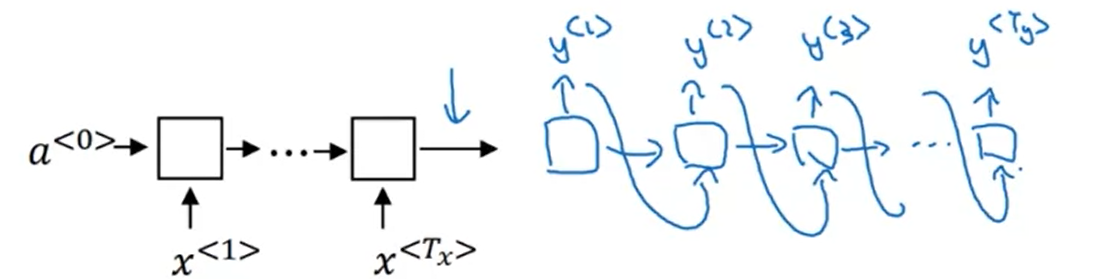This model is a “conditional language model” in the sense that the encoder portion (shown in green) is modelling the probability of the output sentence $y$.

## Image captioning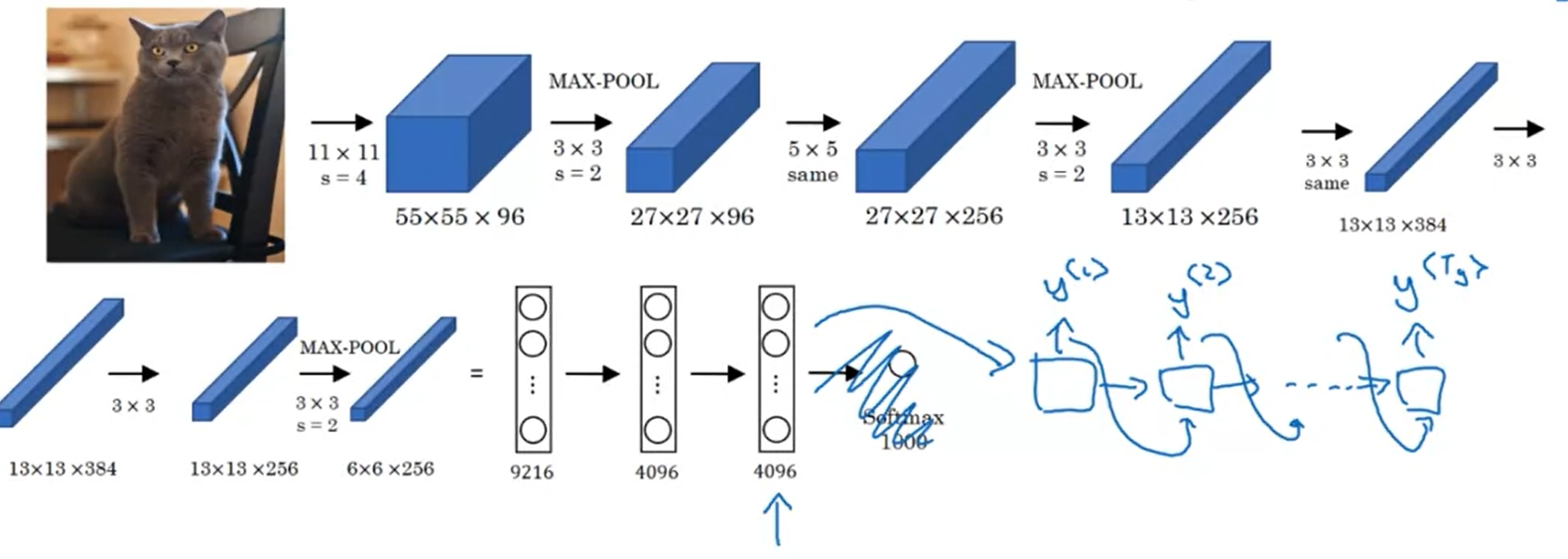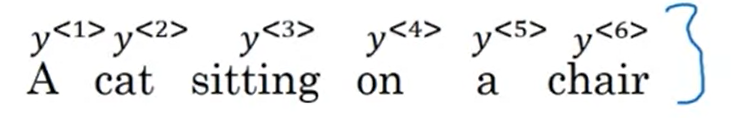## Pick the most likely model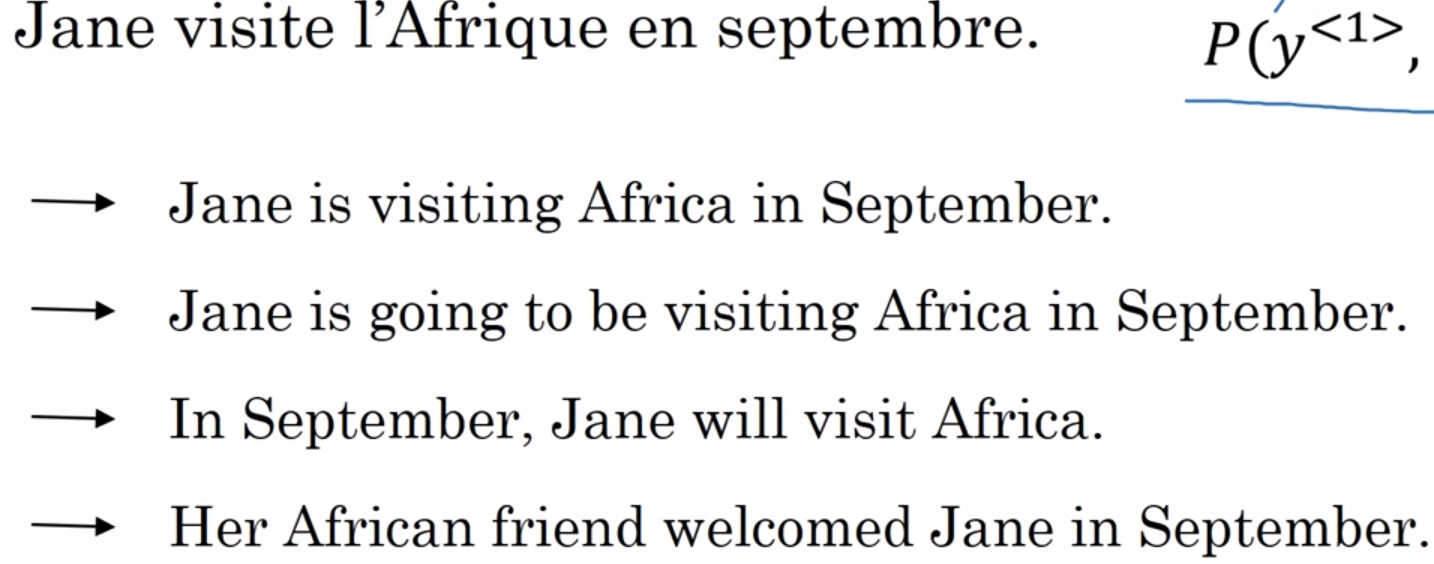Beam width, eg. B = 3

$P(y^{< 1 >} | x)$

Network of copying No., B

When B = 1, it is greedy search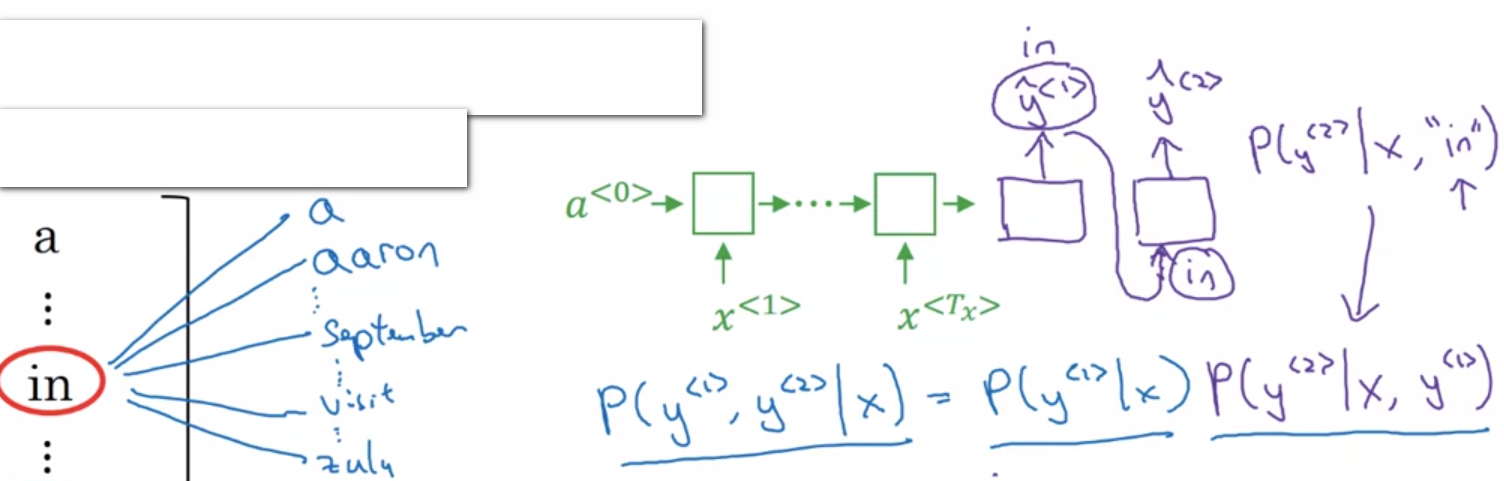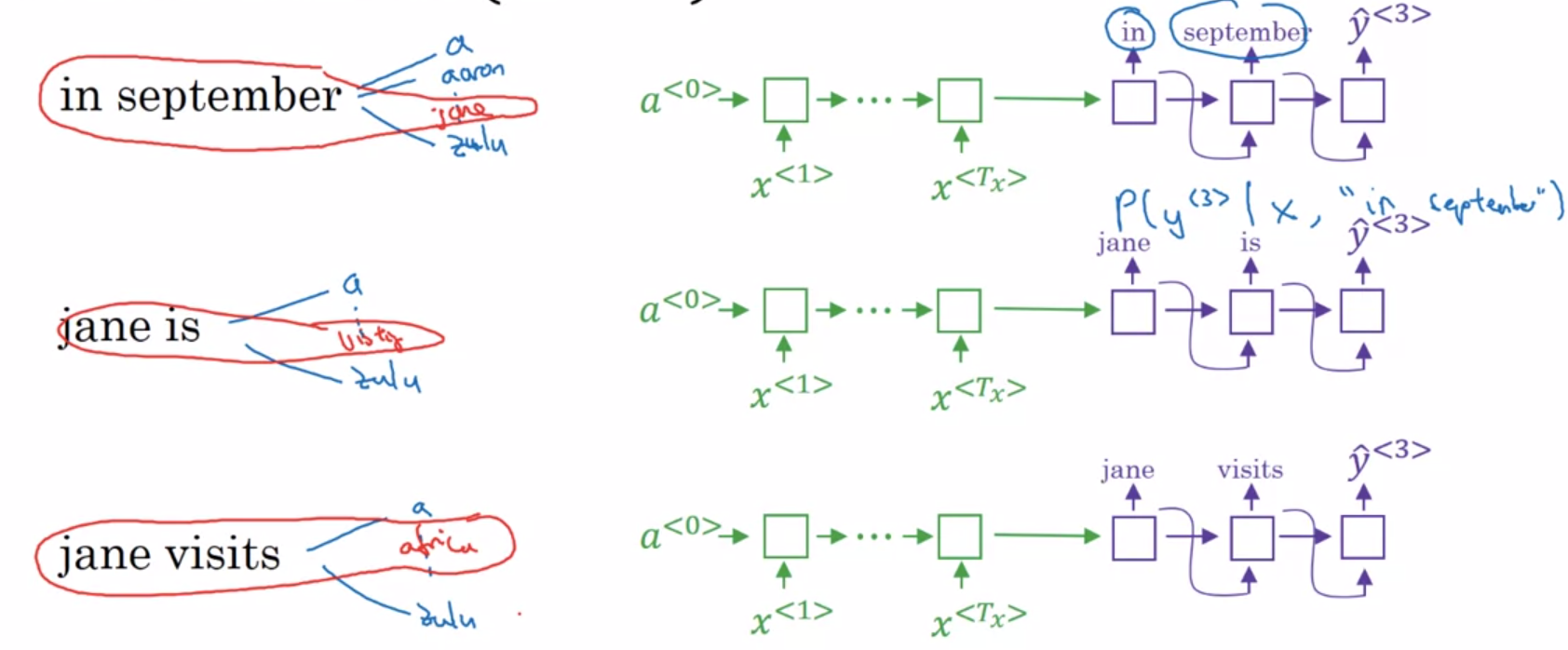Greedy search, pick the first most likely word, and then another. Not works well.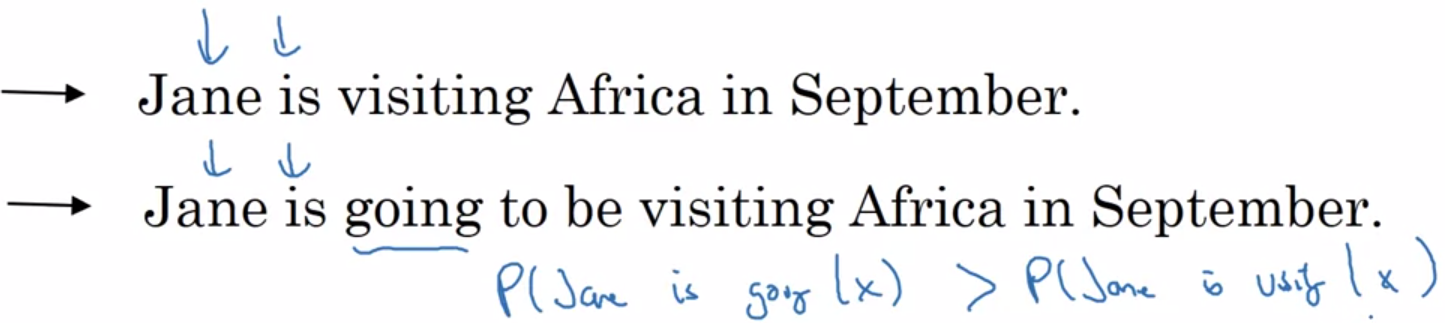Characteristics:

Large B: better result, slower. Small B, worse outcome, faster

Around 10, 100 (product) and about1000, 3000 (specific for research)

Unlike exact search algorithms like BFS (Breadth first search) or DFS (Depth first search), beam search runs faster but is not guaranteed to find the exact maximum for argmax $P(y|x)$.

-[x] Beam search will generally find better solutions (i.e. do a better job maximizing $P(y \mid x)$)

-[x] Beam search will use up more memory.

-[x] Beam search will run more slowly.

## Length normalization

Too small for the floating part representation in your computer to store accurately. The algorithm will tend to output overly short translations in machine translation if we carry out beam search without using sentence normalization.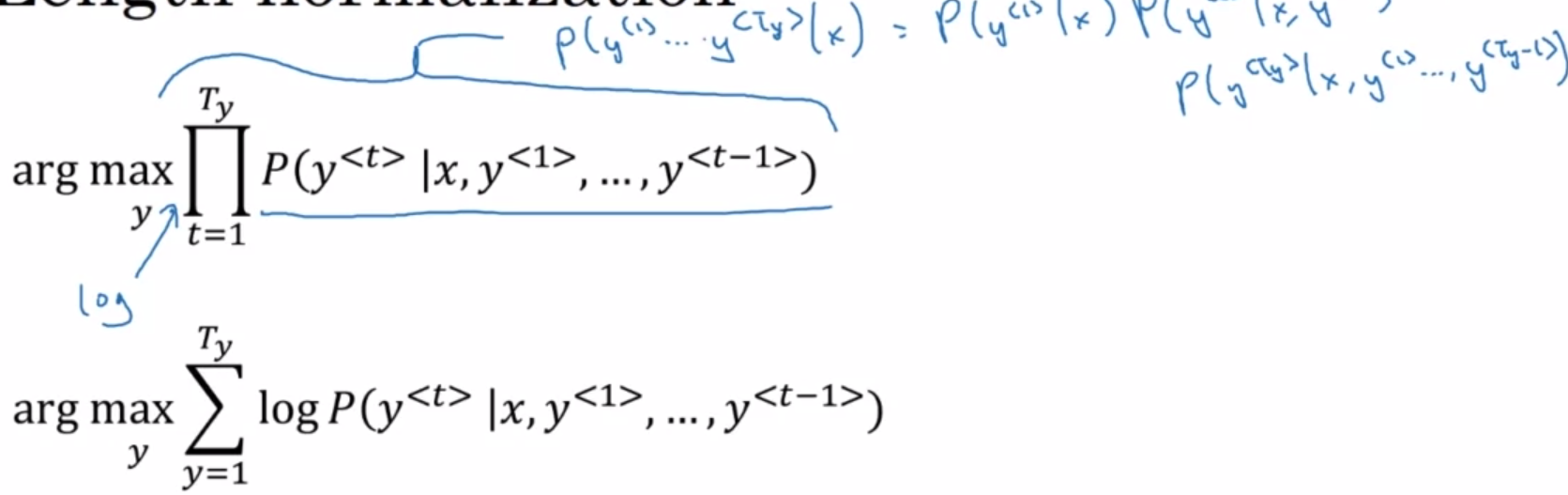## Error analysis

To figure out what fraction of errors is “due to” beam search vs RNN model. You can try to ascribe the error to either the search algorithm or to the objective function or the RNN model that generates the objective function that beam search is supposed to maximize. And through this, you can try to figure out which of these two components is responsible for more errors. And only if you find that beam search is responsible for a lot of mistakes, then maybe is we’re working hard to increase the beam width. P is a possibility !!!

Case 1: $P(y^*|x) > P(\hat{y}|x)$

Conclusion: beam search is at fault

Case 2: $P(y^*|x) \le P(\hat{y}|x)$

Conclusion: RNN model is at fault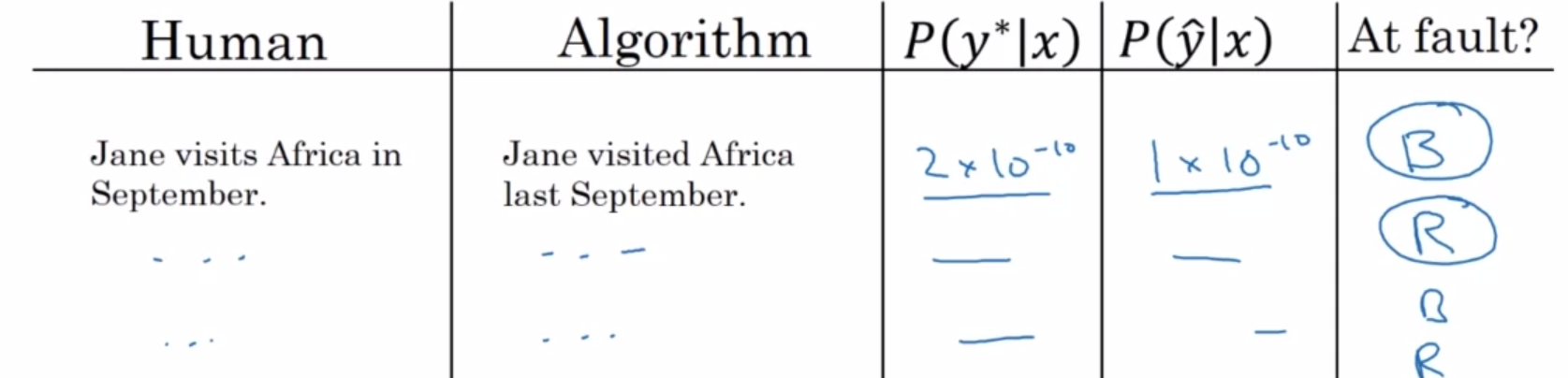## Bleu score

Bleu means bilingual evaluation understudy. To evaluate machine translation, score to measure how good is the machine translation.

Score on bigrams: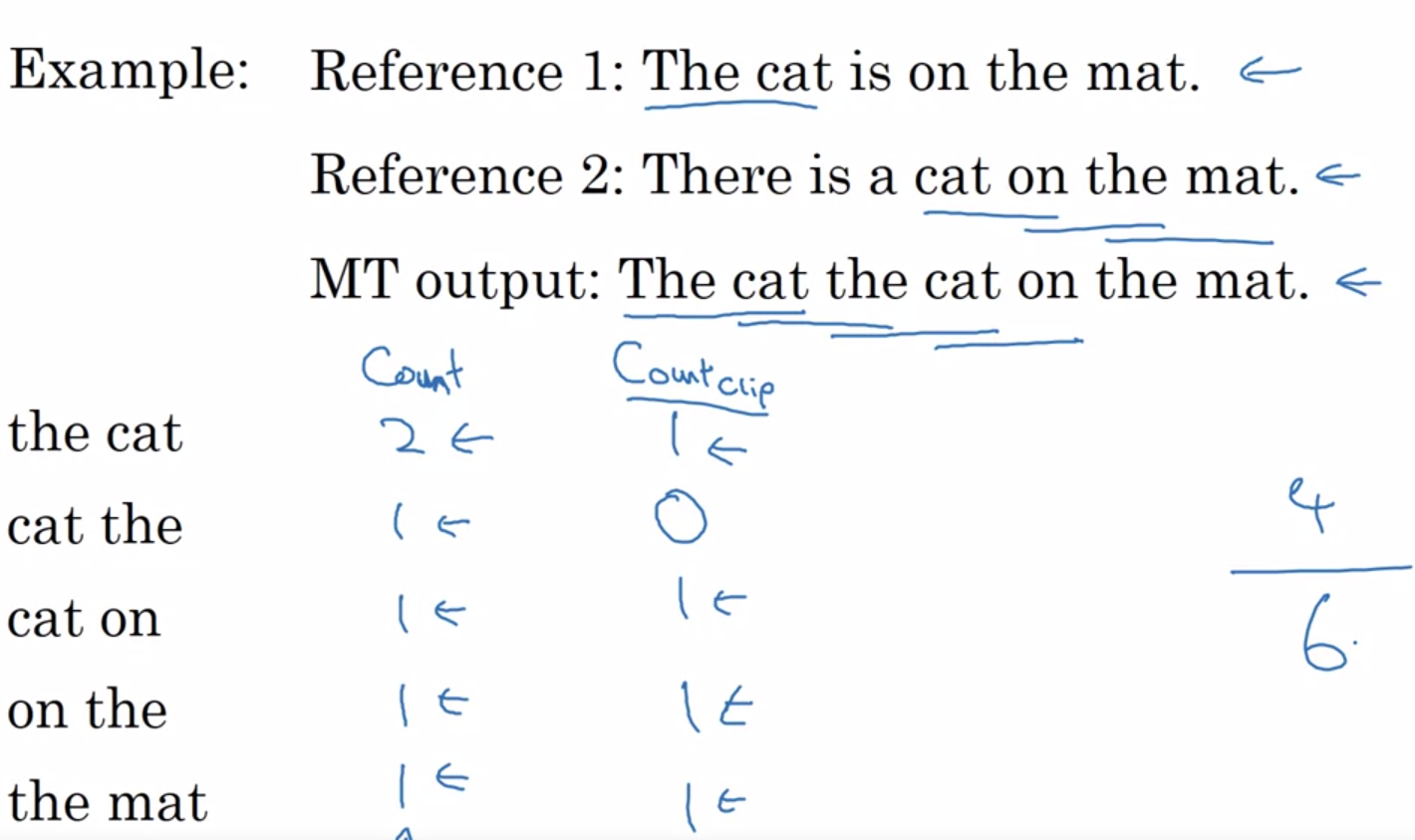Score on unigrams: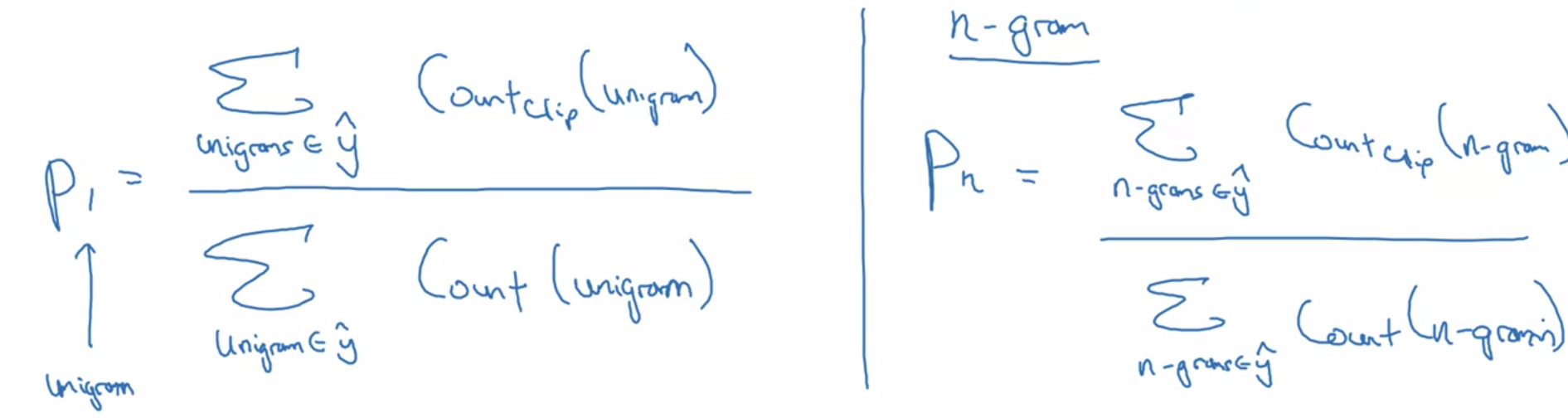Bleu details:

$P_n$ = Blue score on n-grams only

Combined Bleu score: BP $exp(\frac{1}{4} \sum^4_{n=1} P_n)$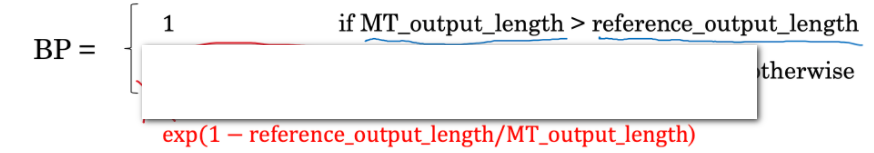## Audio

Use CTC cost for speech recognition. Basic rule: collapse repeated characters not separated by blanks.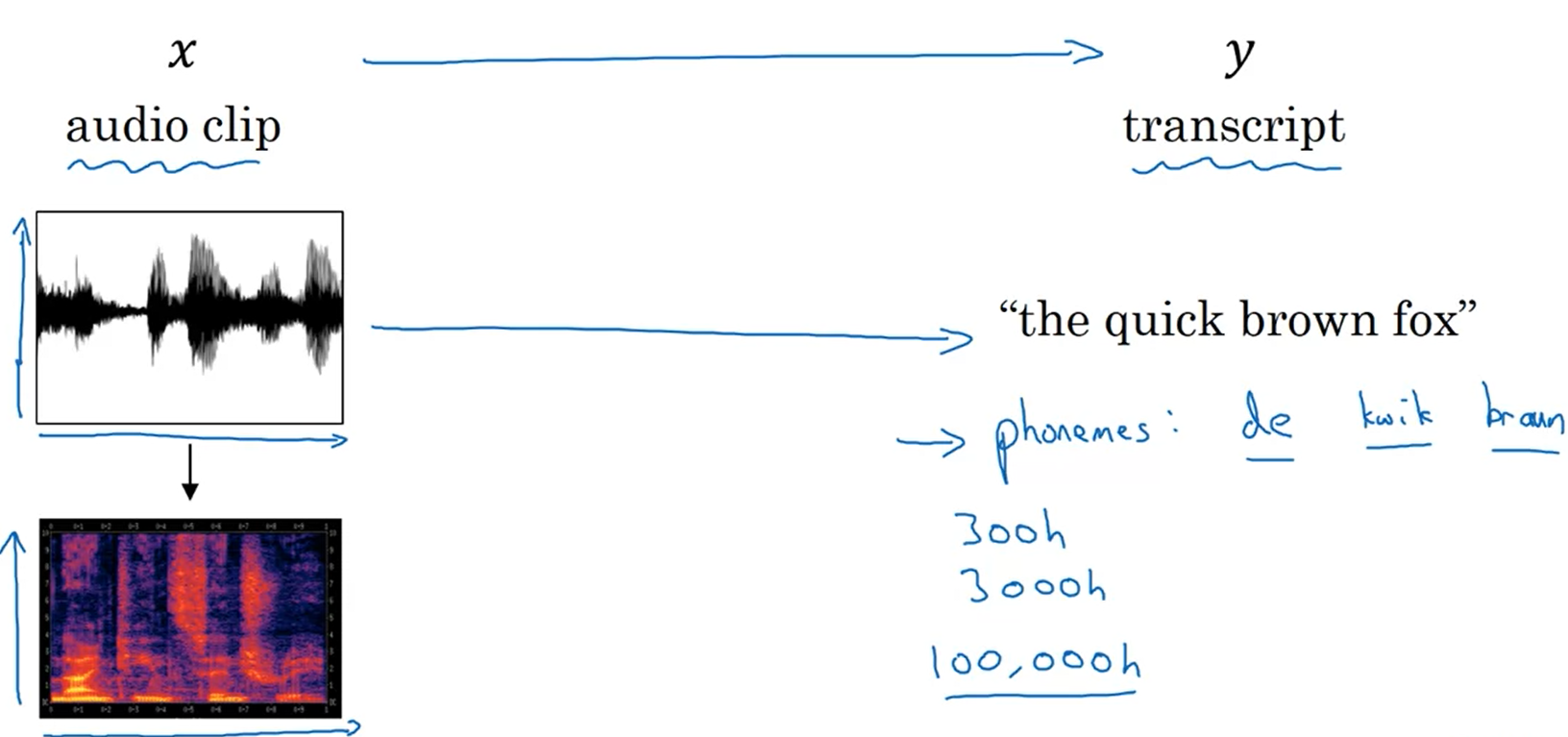Trigger word detection: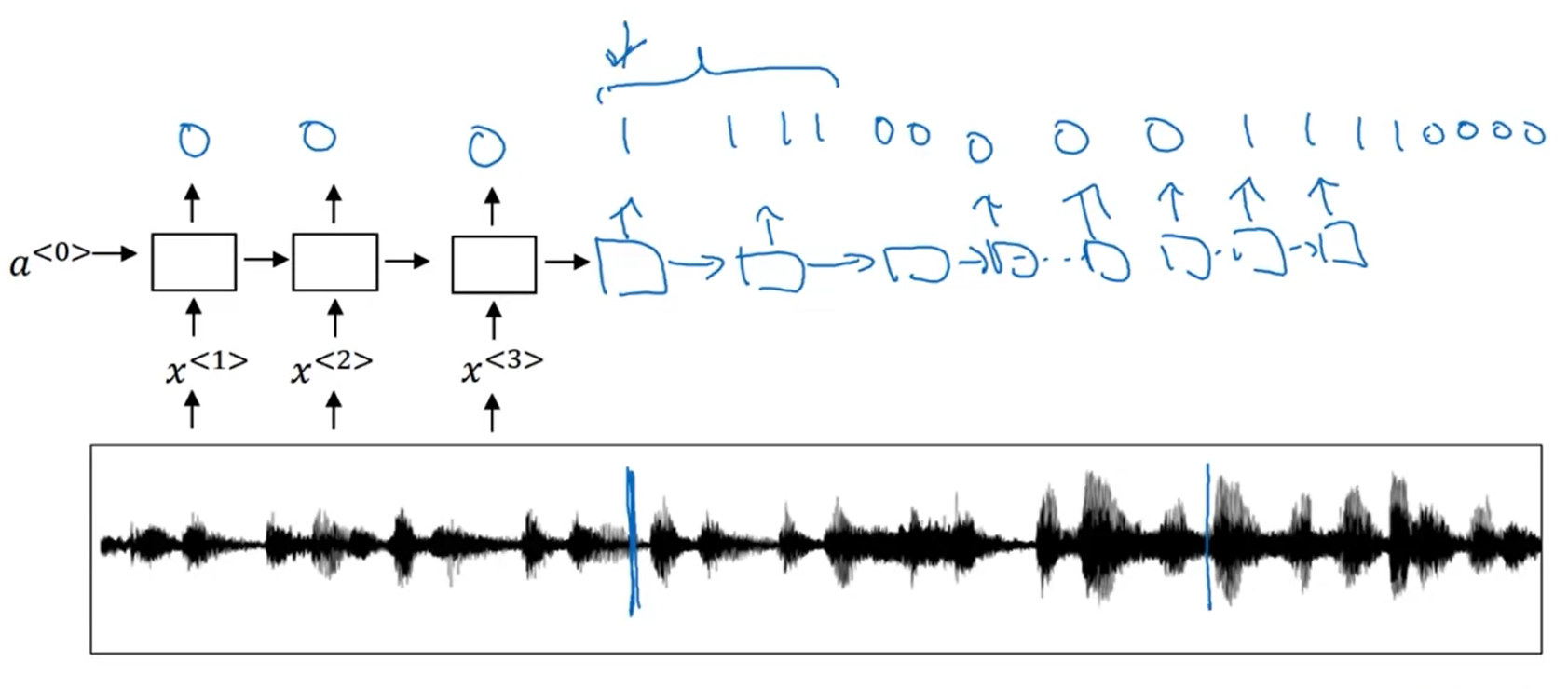In trigger word detection, $x^{< t >}$ is:

-[x] Features of the audio (such as spectrogram features) at time $t$

Whether the trigger word is being said at time tt.

-[ ] Whether someone has just finished saying the trigger word at time $t$.

The t-th input word, represented as either a one-hot vector or a word embedding.

# Reference

 Deeplearning.ai, Sequence Models